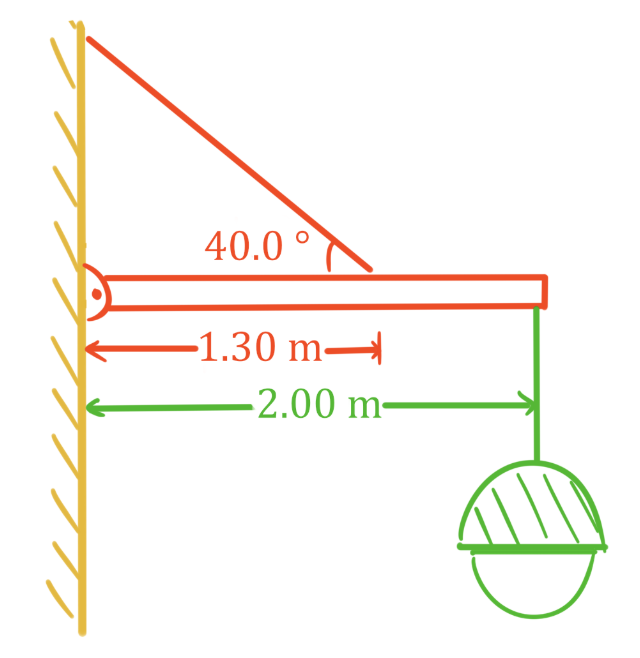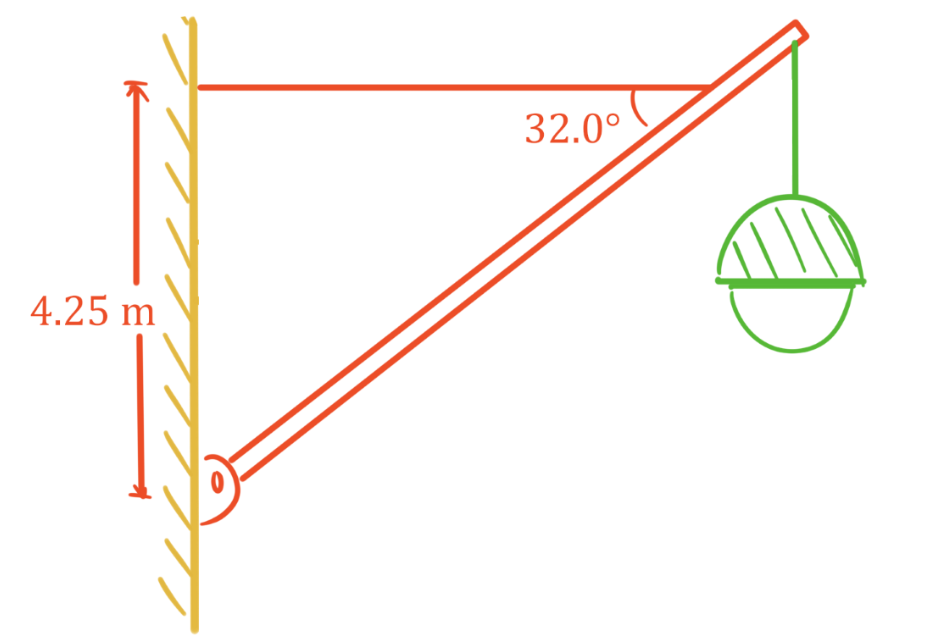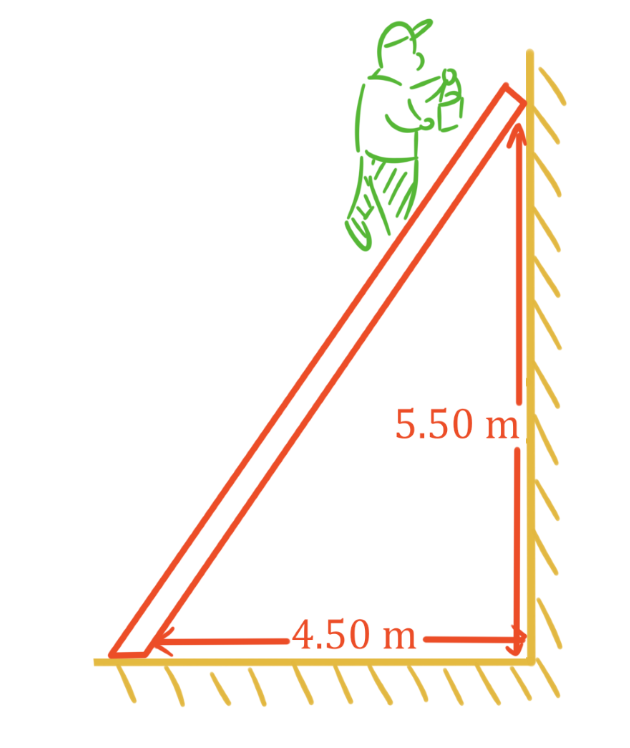# Static equilibrium problems#### Everything You Need in One Place

Homework problems? Exam preparation? Trying to grasp a concept or just brushing up the basics? Our extensive help & practice library have got you covered.#### Learn and Practice With Ease

Our proven video lessons ease you through problems quickly, and you get tonnes of friendly practice on questions that trip students up on tests and finals.#### Instant and Unlimited Help

Our personalized learning platform enables you to instantly find the exact walkthrough to your specific type of question. Activate unlimited help now!##### Examples
###### Lessons
1. Cable and beam in static equilibrium
1. A 20.0 kg lamp is hung from a uniform 12.5 kg beam as shown. Find the tension in the wire, and the horizontal and vertical forces acting on the hinge.2. A 25.0 kg lamp is hung from an 18.0 kg uniform beam as shown. The total length of the beam is 8.50 m. Find the tension in the wire, and the horizontal and vertical forces acting on the hinge.An 80.0 kg painter climbs 85% of the way up a uniform 25.0 kg ladder before it starts to slip backwards along the ground. What is the coefficient of static friction between the ladder and ground? Assume the wall is frictionless.###### Topic Notes

In this lesson, we will learn:

• Solving statics problems using both translational and rotational equilibrium

Notes:

• An object or group of objects that are not moving are in static equilibrium.
• In static equilibrium, the conditions for both translational and rotational equilibrium must be met.
Conditions for Translational Equilibrium

$\Sigma F = 0 N$

or equivalently:

$\Sigma F_{x} = 0 N$ and $\Sigma F_{y} = 0 N$

$\Sigma F:$ sum of all forces, in newtons (N)

$\Sigma F_{x}:$ sum of all force components in x direction, in newtons (N)

$\Sigma F_{y}:$ sum of all force components in y direction, in newtons (N)

Torque

$\tau = F_{\perp}d$

$\tau$: torque, in newton meters (N·m)

$F_{\perp}:$ component of force perpendicular to $d$, in newtons (N)

$d:$ distance from point of rotation, in meters (m)

Conditions for Rotational Equilibrium

$\Sigma \tau = 0$ N·m

or simpler equation:

total $CW$ $\tau$ = total $CCW$ $\tau$

$\Sigma \tau :$ sum of all torques, in newton meters (N·m)

total $CW$ $\tau$: magnitude of all torques in the clockwise direction, in newton meters (N·m)

total $CCW$ $\tau$: magnitude of all torques in the counterclockwise direction, in newton meters (N·m)